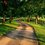# [Differential Geometry] Angle between Curves that meet on the Surface of a Torus

How can we find the angle at which two parametrised differentiable curves $\alpha: I \rightarrow S$ and $\beta : I \rightarrow S$ make on the surface $S$ of a torus? We can take a parametrisation of a torus, $\phi(u,v) = ( \sin u \cos v, \sin u \sin v, \cos u)$.

and let $cos \theta = \frac{\left< \phi_u, \phi_v\right>}{\lvert \phi_u \rvert \lvert \phi_v \rvert} = \frac{F}{\sqrt{EG}} =$. The coefficients of the first fundamental form can be calculated as follows: The differential of the map $\phi$ with respect to $u$ is $\frac{\partial \phi}{ \partial u}$, written as $\phi_u$ for convenience, $\phi_u = ( \cos u \cos v, \cos u \sin v, -\sin u).$ Similarly, $\phi_v = ( -\sin u \sin v, \sin u \cos v , 0).$ Hence $E= \left< \phi_u, \phi_u \right> = \cos^2(u) + \sin^2(u) = 1$, $F= \left< \phi_u, \phi_v \right> = 0$, and $G= \left< \phi_v, \phi_v \right> = \sin^2(u).$ Hence the angle at which the two coordinate curves of the torus meet are: $\frac{F}{\sqrt{EG} = 0.}$ Now the coordinate curves of a parametrisation are orthogonal if and only if $F(u,v) = 0$ for all $(u,v)$. And such a parametrisation is called an orthogonal projection. Therefore, we see that the above parametrisation $\phi$ is an orthogonal projection.

The angle at which the two curves meet at $t= t_0$ can be expressed as $cos \theta_1 = \frac{ \left< \alpha'(t_0), \beta' (t_0) \right>}{\lvert \alpha'(t_0) \rvert \lvert \alpha'(t_0) \rvert}$ where $\alpha ' (t) = \phi_u a + \phi_v b$ and $\beta '(t) = \phi_u c + \phi_v d$. Hence, we have

$\frac {E (ac) + F (bc) + F (ad) + G ( bd)}{\sqrt{a^2 + b^2\sin^2(u)} \sqrt{c^2 + d^2\sin^2(u) }} =\frac { ac+ \sin^2(u)( bd)}{\sqrt{(a^2 + b^2\sin^2(u))(c^2 + d^2\sin^2(u)) }} .$Note by Bright Glow
3 years, 1 month ago

This discussion board is a place to discuss our Daily Challenges and the math and science related to those challenges. Explanations are more than just a solution — they should explain the steps and thinking strategies that you used to obtain the solution. Comments should further the discussion of math and science.

When posting on Brilliant:

• Use the emojis to react to an explanation, whether you're congratulating a job well done , or just really confused .
• Ask specific questions about the challenge or the steps in somebody's explanation. Well-posed questions can add a lot to the discussion, but posting "I don't understand!" doesn't help anyone.
• Try to contribute something new to the discussion, whether it is an extension, generalization or other idea related to the challenge.
• Stay on topic — we're all here to learn more about math and science, not to hear about your favorite get-rich-quick scheme or current world events.

MarkdownAppears as
*italics* or _italics_ italics
**bold** or __bold__ bold
- bulleted- list
• bulleted
• list
1. numbered2. list
1. numbered
2. list
Note: you must add a full line of space before and after lists for them to show up correctly
paragraph 1paragraph 2

paragraph 1

paragraph 2

[example link](https://brilliant.org)example link
> This is a quote
This is a quote
    # I indented these lines
# 4 spaces, and now they show
# up as a code block.

print "hello world"
# I indented these lines
# 4 spaces, and now they show
# up as a code block.

print "hello world"
MathAppears as
Remember to wrap math in $$ ... $$ or $ ... $ to ensure proper formatting.
2 \times 3 $2 \times 3$
2^{34} $2^{34}$
a_{i-1} $a_{i-1}$
\frac{2}{3} $\frac{2}{3}$
\sqrt{2} $\sqrt{2}$
\sum_{i=1}^3 $\sum_{i=1}^3$
\sin \theta $\sin \theta$
\boxed{123} $\boxed{123}$

## Comments

There are no comments in this discussion.

×

Problem Loading...

Note Loading...

Set Loading...